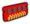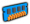# Experiment: Electricity

The Electricity experiment is one of 12 experiments in the laboratory, available at laboratory tier 2.

The goal of the Electricity Experiment is to raise the amperage.

## Mechanic

Each coil (battery) starts with 1 wrap (cell), with wraps capping at 20 per coil and coils capping at 5.

Each wrap adds 1V on the coil it's on.

The total voltage is calculated with the product of voltages of all coils.

Each wrap's material can be upgraded to reduce it's resistance.

The resistance of a coil is the average of the resistances of all wraps in that coil.

Total resistance is the average of the resistances of all coils

Amperage is calculated with Voltage(V) / Resistance(Ohm)

### Prestige

Prestige resets all coils and wraps, you have to choose between the following bonuses:

• +30% V: Increases voltage power by 30% (multiplicative)
• -20% Ohm: Reduces electrical resistance by 20% (multiplicative)

## Rewards

Amperage Reward
5AElectricity Taste
12ALightning
30AOvercharge
150ATransformator
1000AShock ward
2lvl*100A +(lvl+1)% Electricity damage
8lvl*150A +(lvl+1)% Electricity resistance